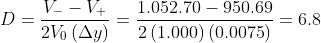Scan QR code or get instant email to install app

Question:

# An 8%, semiannual pay, option-free corporate bond that is selling at par has ten years to maturity. What is the approximate modified duration of the bond based on a 75 basis point change (up or down) in rates?

A 6.8.
explanation

1212First calculate V- and V+, the bonds value at 7.25% and 8.75% yields to maturity. The bond values are 1,052.70 and 950.69, respectively:
N = 20; I/Y = 7.25 / 2 = 3.625; PMT = 40; FV = 1,000; CPT PV = -1052.70
N = 20; I/Y = 8.75 / 2 = 4.375; PMT = 40; FV = 1,000; CPT PV = -950.69